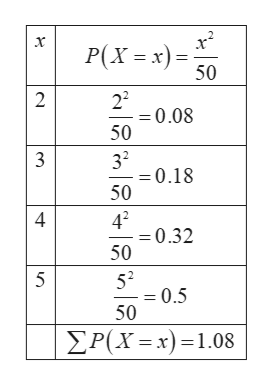# given below are tables or formulas which might represent a discrete probability distribution. If it could ne a discrete probability distribution write "yes". If the information given in the table or formula could not represent a discrete probability, write "no" AND explain why it can't represent a discrete probability? d)x12322P (X = x).1.2.3.4 e)x-1012P (X = x).4.3.2.1 f) P (X = x) = x^2 / 50 ; x = 2, 3, 4 , 5 g) P (X = x) = x / 20 ; x = 2, 3, 4, 5, 6

Question

given below are tables or formulas which might represent a discrete probability distribution. If it could ne a discrete probability distribution write "yes". If the information given in the table or formula could not represent a discrete probability, write "no" AND explain why it can't represent a discrete probability?

d)

 x 1 2 3 22 P (X = x) 0.1 0.2 0.3 0.4

e)

 x -1 0 1 2 P (X = x) 0.4 0.3 0.2 0.1

f)

P (X = x) = x^2 / 50 ; x = 2, 3, 4 , 5

g) P (X = x) = x / 20 ; x = 2, 3, 4, 5, 6

check_circle

Step 1

Discrete probability distribution:

If the given random variable is discrete random variable, then its probability distribution is known as discrete probability distribution.

The conditions for discrete probability distribution is given below:

The probability value is between 0 and 1.

Sum of all probabilities is 1.

(d)

The sum of probabilities is obtained as follows:

Step 2

Here, the sum of probabilities is equal to 1. Hence, the condition for

discrete probability distribution is satisfied. Hence, the given table represent the discrete probability distribution.

(e)

The sum of probabilities is obtained as follows:

Step 3

Here, the sum of probabilities is equal to 1. Hence, the condition for

discrete probability distribution is satisfied. Hence, the given table r...help_outlineImage Transcriptionclosex P(Xx) 50 22 0.08 50 2 32 -0.18 50 3 42 =0.32 50 4 52 = 0.5 50 5 P(X x)1.08 fullscreen

### Want to see the full answer?

See Solution

#### Want to see this answer and more?

Solutions are written by subject experts who are available 24/7. Questions are typically answered within 1 hour.*

See Solution
*Response times may vary by subject and question.
Tagged in

### Other